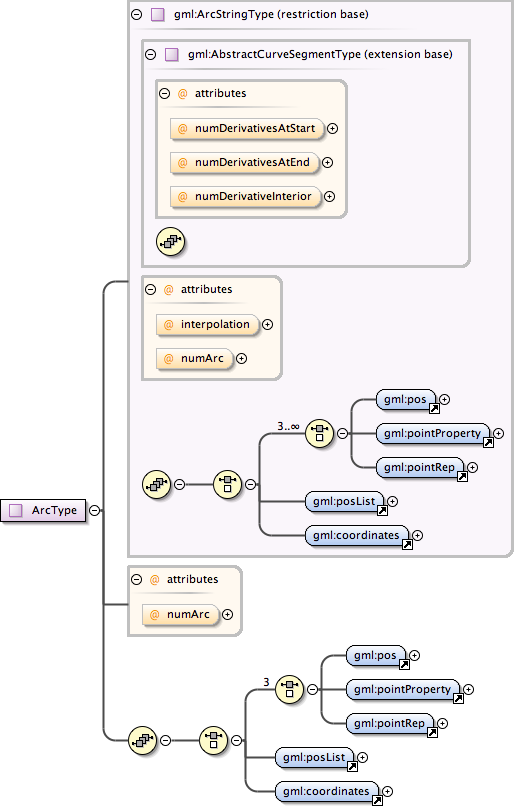### Showing:

 Annotations Attributes Diagrams Properties Source Used by
Complex Type gml:ArcType
Namespace http://www.opengis.net/gml
Annotations
 `An Arc is an arc string with only one arc unit, i.e. three control points.`
DiagramType restriction of gml:ArcStringType
Type hierarchy
Used by
 Element gml:Arc Complex Type gml:CircleType
Model (gml:pos | gml:pointProperty | gml:pointRep | gml:posList | gml:coordinates)
Children gml:coordinates, gml:pointProperty, gml:pointRep, gml:pos, gml:posList
Attributes
QName Type Fixed Default Use Annotation
interpolation gml:CurveInterpolationType circularArc3Points optional
 ```The attribute "interpolation" specifies the curve interpolation mechanism used for this segment. This mechanism uses the control points and control parameters to determine the position of this curve segment. For an ArcString the interpolation is fixed as "circularArc3Points".```
numArc integer 1 optional
 `An arc is an arc string consiting of a single arc, the attribute is fixed to "1".`
numDerivativeInterior integer 0 optional
 ```The attribute "numDerivativesInterior" specifies the type of continuity that is guaranteed interior to the curve. The default value of "0" means simple continuity, which is a mandatory minimum level of continuity. This level is referred to as "C 0 " in mathematical texts. A value of 1 means that the function and its first derivative are continuous at the appropriate end point: "C 1 " continuity. A value of "n" for any integer means the function and its first n derivatives are continuous: "C n " continuity. NOTE: Use of these values is only appropriate when the basic curve definition is an underdetermined system. For example, line string segments cannot support continuity above C 0 , since there is no spare control parameter to adjust the incoming angle at the end points of the segment. Spline functions on the other hand often have extra degrees of freedom on end segments that allow them to adjust the values of the derivatives to support C 1 or higher continuity.```
numDerivativesAtEnd integer 0 optional
 ```The attribute "numDerivativesAtEnd" specifies the type of continuity between this curve segment and its successor. If this is the last curve segment in the curve, one of these values, as appropriate, is ignored. The default value of "0" means simple continuity, which is a mandatory minimum level of continuity. This level is referred to as "C 0 " in mathematical texts. A value of 1 means that the function and its first derivative are continuous at the appropriate end point: "C 1 " continuity. A value of "n" for any integer means the function and its first n derivatives are continuous: "C n " continuity. NOTE: Use of these values is only appropriate when the basic curve definition is an underdetermined system. For example, line string segments cannot support continuity above C 0 , since there is no spare control parameter to adjust the incoming angle at the end points of the segment. Spline functions on the other hand often have extra degrees of freedom on end segments that allow them to adjust the values of the derivatives to support C 1 or higher continuity.```
numDerivativesAtStart integer 0 optional
 ```The attribute "numDerivativesAtStart" specifies the type of continuity between this curve segment and its predecessor. If this is the first curve segment in the curve, one of these values, as appropriate, is ignored. The default value of "0" means simple continuity, which is a mandatory minimum level of continuity. This level is referred to as "C 0 " in mathematical texts. A value of 1 means that the function and its first derivative are continuous at the appropriate end point: "C 1 " continuity. A value of "n" for any integer means the function and its first n derivatives are continuous: "C n " continuity. NOTE: Use of these values is only appropriate when the basic curve definition is an underdetermined system. For example, line string segments cannot support continuity above C 0 , since there is no spare control parameter to adjust the incoming angle at the end points of the segment. Spline functions on the other hand often have extra degrees of freedom on end segments that allow them to adjust the values of the derivatives to support C 1 or higher continuity.```
Source
 ``` An Arc is an arc string with only one arc unit, i.e. three control points. GML supports two different ways to specify the control points of a curve segment. 1. A sequence of "pos" (DirectPositionType) or "pointProperty" (PointPropertyType) elements. "pos" elements are control points that are only part of this curve segment, "pointProperty" elements contain a point that may be referenced from other geometry elements or reference another point defined outside of this curve segment (reuse of existing points). 2. The "posList" element allows for a compact way to specifiy the coordinates of the control points, if all control points are in the same coordinate reference systems and belong to this curve segment only. The number of direct positions in the list must be three. Deprecated with GML version 3.1.0. Use "pointProperty" instead. Included for backwards compatibility with GML 3.0.0. Deprecated with GML version 3.1.0. Use "posList" instead. An arc is an arc string consiting of a single arc, the attribute is fixed to "1". ```
Schema location http://schemas.opengis.net/gml/3.1.1/base/geometryPrimitives.xsd
Attribute gml:ArcType / @numArc
Namespace No namespace
Annotations
 `An arc is an arc string consiting of a single arc, the attribute is fixed to "1".`
Type integer
Properties
 use: optional fixed: 1
Used by
 Complex Type gml:ArcType
Source
 ``` An arc is an arc string consiting of a single arc, the attribute is fixed to "1". ```
Schema location http://schemas.opengis.net/gml/3.1.1/base/geometryPrimitives.xsd